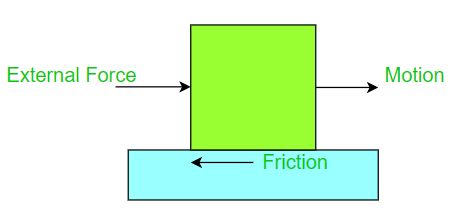What is Friction?

• Last Updated : 21 Jul, 2021

When you’re freezing, what’s the first thing you do? There are two options here: either wrap yourself in a warm blanket or massage your hands together to warm yourself. The issue now is, Why? When we touch our hands together, how do they get warm? Friction is generated by rubbing our hands together, which produces heat. This gives us a warm feeling.

When we throw a ball on the floor, it begins to move quickly. However, no force should be operating in the direction of motion, and the ball should continue to roll according to Newton’s first law, but this does not occur. Instead, the ball comes to a halt after a given distance, indicating that a force is operating on it. So, what exactly is ‘Friction?’ Why is it required? Let’s take a closer look at it.

What is Friction?

Friction is a force that opposes the motion of one item sliding or rolling relative to another.

The electromagnetic attraction between the charged particles of two contacting surfaces causes it. We come across and use it every day as items come into contact with one another. Even though it always functions in the opposite direction of how an object desires to slide.

For example, when we wish to stop or slow down, we use automobile brakes because the friction generated between the brakes and the wheels causes the car to slow down or stop.Friction gives the necessary traction for walking without slipping. In most situations, friction is beneficial. They do, however, provide a significant amount of resistance to the proposal. Furthermore, roughly 20% of an automobile’s engine power is used to overcome frictional forces in the moving parts. Let’s have a look at some of these characteristics in the following section.

Factors affecting Friction

The force of friction may change as a result of the following factors:

• When two rough surfaces come into contact, the degree of friction between them is high due to the excessive interlocking of rough surfaces.
• Because there is less interlocking between smooth surfaces when two smooth surfaces are in touch, the degree of friction between them is low.
• It is also affected by the object’s weight or the amount of force it exerts on the surface.

What causes Friction?

The irregularities on the two surfaces in contact produce friction. As a result, as one object passes over another, the irregularities on the surface become intertwined, causing friction. The rougher the surface, the more irregularities there will be and the friction will be greater.

Types of Friction

There are primarily two types of friction:

1. Dry Friction: It takes place between solid surfaces. It has four types.

• Static Friction
• Kinetic Friction
• Rolling Friction
• Sliding Friction

2. Fluid Friction: It takes place in liquids and gases.

Effects of Friction

• It also results in a loss of power.
• We can walk, run, play, and so forth due to friction.
• It generates heat, which may be used to warm sections of an item or ourselves.
• During any operation, it generates noise.

Application of Friction

• Friction is created by the movement of pistons in a cylinder.
• When matchsticks are lit, friction comes into play.
• Because there is friction between the pen and the board, writing on books and boards is feasible.

Laws of Friction

The following are the five laws of friction:

• The friction between the moving item and the normal force is proportionate and perpendicular.
• Friction exists regardless of the area of contact as long as there is one.
• The object’s friction is determined by the type of surface it comes into contact with.
• The static friction coefficient is higher than the kinetic friction coefficient.
• Velocity has no effect on kinetic friction.

Sample Problems

Problem 1: A girl runs her toy car on a dry marble floor, wet marble floor, newspaper, and towel spread on the floor. Arrange the force of friction acting on the car on different surfaces in increasing order.

The friction force between two surfaces is determined by their nature. If the surfaces in touch are rougher, friction will be higher. Because the wet marble floor is the least abrasive of the three, it will be subjected to the least amount of force. So, in order of roughness, wet marble floor, dry marble floor, newspaper, and towel are the roughest. As a result, the force will be in the same sequence.

Problem 2: Can friction be zero?

No, it is impossible to have zero friction because every surface will have minor irregularities no matter how lubricated.

Problem 3: What is the relation between friction and gravity?

The formula for frictional force is given as:

F = μ N

where μ is the coefficient of friction  and N is the Normal force.

Here,

N = m g

where g is acceleration due to gravity or strength of gravity.

Normal force is a perpendicular force applied to the surface to the body. It is acted on the body by surface under the influence of gravity on the body in the downward direction.

Gravity is the downward acceleration experienced by anybody on the surface of any planet. This is responsible for weight experienced by the body. On a flat surface weight and Normal are opposite in direction. The surface in contact produces frictional force.

Friction is opposite to the direction of push applied on the body to make it move. If gravity is very strong it becomes harder to slide a body on a surface because of increased force of friction.

Problem 4: When we apply brakes in the car, it retards the motion; although it is an internal force. Why?